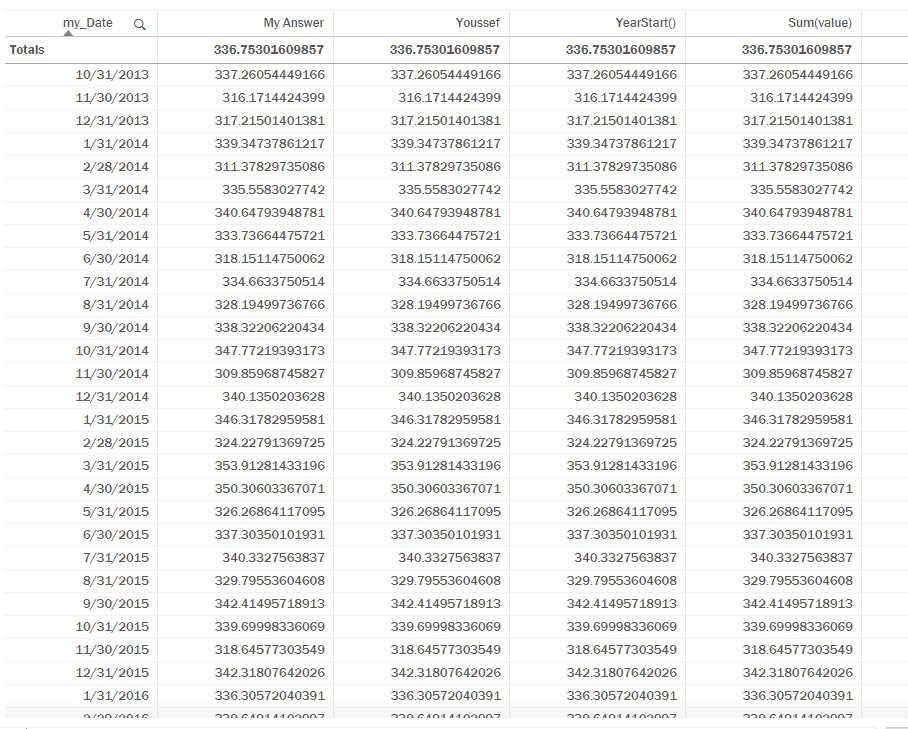# Qlik Sense App Development

Announcements
Qlik Analytics Tour 2020 Online. Begins August 10th. Register Today
cancel
Showing results for
Did you mean:
HighlightedContributor III

## FY YTD Sum

I'm attempting to create a Fiscal Year to date sum but I'm struggling to have my start date recognized by qlik sense.

Our Fiscal Year starts 1 October so I'm trying the following:

```

SUM({\$<

my_Date={'>=\$(=Date#((Year(Max(my_Date))-1)&''-10-01'', ''YYYY-MM-DD''))<=\$(=Max(my_Date))'}>}

my_Value

)

```

But this sums my entire date range until the current date. I created a table to check what was happening

```

'>=\$(=Date#((Year(Max(my_Date))-1)&''-10-01'', ''YYYY-MM-DD''))<=\$(=Max(my_Date))'

```

Which produces the following:

>=<6/30/2018

Basically, it doesn't create my starting date. However, If I just put

```

=Date#((Year(Max(my_Date))-1)&'-10-01', 'YYYY-MM-DD')

```

i.e. not inside a string literal but just as a measure, the correct date appears.

So I'm not sure why I cannot select the proper start date in my set analysis. The my_Date column is all dates, one a month each at month end.

idxDate
14/30/2018
25/31/2018
36/30/2018

Docs I've been trying to use:

YTQ, QTD, MTD and WTD

Set Analysis for Rolling Periods

https://help.qlik.com/en-US/sense/September2018/Subsystems/Hub/Content/Sense_Hub/Scripting/use-quote...

EDIT:

How do I create a Year to Date Measure?

It appears I can do this by month but not if I roll up to the quarter level because the number you move above is incorrect.

Tags (4)
5 Replies
HighlightedChampion

## Re: FY YTD Sum

Hi,

try it like this:

SUM({\$<

my_Date={'>=\$(=Date(Date#((Year(Max(my_Date))-1)&''-10-01'', ''YYYY-MM-DD''),'M/DD/YYYY'))<=\$(=Max(my_Date))'}>}

my_Value

)

HighlightedContributor III

## Re: FY YTD SumHere are the results fro the following methods:

SUM({\$<

my_Metric={'cost'},

my_Metric_Component={'Total Costs'},

my_Service={'food'},

my_Date={'>=\$(=Date#((Year(Max(my_Date))-1)&''-10-01'', "YYYY-MM-DD"))<=\$(=Max(my_Date))'}>}

my_Value

)

SUM({\$<

my_Metric={'cost'},

my_Metric_Component={'Total Costs'},

my_Service={'food'},

my_Date={'>=\$(=Date(Date#((Year(Max(my_Date))-1)&''-10-01'', ''YYYY-MM-DD''),`M/DD/YYYY`))<=\$(=Max(my_Date))'}>}

my_Value

)

YearStart():

SUM({\$<

my_Metric={'cost'},

my_Metric_Component={'Total Costs'},

my_Service={'food'},

my_Date={'>=\$(=MIN(YearStart(my_Date, 0, 10)))<=\$(=Max(my_Date))'}>}

my_Value

)

Sum(value):

SUM({\$<

my_Metric={'cost'},

my_Metric_Component={'Total Costs'},

my_Service={'food'}

>}

my_Value

)

As you can see, no matter what I do with my date column, I always get the same results. In the last one I remove the date filter all together and get the same thing.

HighlightedContributor III

## Re: FY YTD Sum

The following solution works if every data point is shown, i.e. no roll-up

IF( month(my_Date) = 10,

Sum({\$<my_Service={'food'},my_Metric_Component={'Total Costs'}>}my_Value)

,

RangeSum(Above( Sum({\$<my_Service={'food'}, my_Metric_Component={'Total Costs'}>}my_Value) , 0, month(my_Date) - 10 + IF( Month(my_Date) < 10, 13, 1)))

)

However, If I try to show data by Quarter instead of by month this does not work. Partial solution..

HighlightedChampion

## Re: FY YTD Sum

Would you be able to share a QVF file that shows the issue ?

HighlightedContributor III